• 基于MATLAB图像处理中值滤波均值滤波以及高斯滤波的实现与对比: a) 中值滤波法是一种非线性平滑技术，它将每一像素点的灰度值设置为该点某邻域窗口内的所有像素点灰度值的中值. b) 均值滤波是典型的线性滤波算法...
• Lena椒盐噪声图片： # -*- coding: utf-8 -*- """ Created on Sat Oct 14 22:16:47 2017 @author: Don """ from tkinter import * from skimage import io import numpy as np im=io.imread('lena_sp.jpg', as_...
• 关于数字图像处理均值滤波可以去除高斯噪声，但是均值滤波会使图像变得模糊，中值滤波能有效滤除椒盐噪声。
• 用高斯滤波、均值滤波中值滤波，双边滤波滤波进行去噪处理，这四种滤波是在添加高斯噪声的基础上，分别得到信噪比，通过对比信噪比值，最终得到那种方式处理效果最好。并且源码可以在不同的卷积核滤波器大小、添加...Matlab
• 题目均值滤波和中值滤波 在自己的证件照中加入椒盐噪声高斯噪声 分别用3*35*57*7 的均值滤波器中值滤波器进行滤波 处理过程 1. 用imnoise函数在图像中分别加入椒盐噪声和高斯噪声 2. 均值滤波用fspecial函数...
• 基于MATLAB图像处理中值滤波均值滤波以及高斯滤波的实现与对比作者：lee神1.背景知识中值滤波法是一种非线性平滑技术，它将每一像素点的灰度值设置为该点某邻域窗口内的所有像素点灰度值的中值.中值滤波是基于...

基于MATLAB图像处理的中值滤波、均值滤波以及高斯滤波的实现与对比

作者：lee神

1.背景知识

中值滤波法是一种非线性平滑技术，它将每一像素点的灰度值设置为该点某邻域窗口内的所有像素点灰度值的中值.

中值滤波是基于排序统计理论的一种能有效抑制噪声的非线性信号处理技术，中值滤波的基本原理是把数字图像或数字序列中一点的值用该点的一个邻域中各点值的中值代替，让周围的像素值接近的真实值，从而消除孤立的噪声点。

方法是用某种结构的二维滑动模板，将板内像素按照像素值的大小进行排序，生成单调上升(或下降)的为二维数据序列。二维中值滤波输出为g(x,y)=med{f(x-k,y-l),(k,l∈W)}，其中，f(x,y)，g(x,y)分别为原始图像和处理后图像。W为二维模板，通常为3*3，5*5区域，也可以是不同的的形状，如线状，圆形，十字形，圆环形等。

2

4

8

1

3

9

5

7

6

g(x,y)=med{f(x-k,y-l),(k,l∈W)}

g =med[2,4,8;1,3,9;5,7,6] = 5

中值滤波后的结果：

5

均值滤波是典型的线性滤波算法，它是指在图像上对目标像素给一个模板，该模板包括了其周围的临近像素(以目标像素为中心的周围8个像素，构成一个滤波模板，即去掉目标像素本身)，再用模板中的全体像素的平均值来代替原来像素值。

均值滤波也称为线性滤波，其采用的主要方法为邻域平均法。线性滤波的基本原理是用均值代替原图像中的各个像素值，即对待处理的当前像素点(x，y)，选择一个模板，该模板由其近邻的若干像素组成，求模板中所有像素的均值，再把该均值赋予当前像素点(x，y)，作为处理后图像在该点上的灰度g(x，y)，即g(x，y)=1/m ∑f(x，y)m为该模板中包含当前像素在内的像素总个数。

均值滤波本身存在着固有的缺陷，即它不能很好地保护图像细节，在图像去噪的同时也破坏了图像的细节部分，从而使图像变得模糊，不能很好地去除噪声点。

2

4

8

1

3

9

5

7

6

g(x，y)=1/m ∑f(x，y)

g = (1/8)*(2+4+8+1+9+5+7+6)= 5

均值滤波后的结果：

5

高斯滤波是一种线性平滑滤波，适用于消除高斯噪声，广泛应用于图像处理的减噪过程。通俗的讲，高斯滤波就是对整幅图像进行加权平均的过程，每一个像素点的值，都由其本身和邻域内的其他像素值经过加权平均后得到。高斯滤波的具体操作是：用一个模板(或称卷积、掩模)扫描图像中的每一个像素，用模板确定的邻域内像素的加权平均灰度值去替代模板中心像素点的值。

2.MATLAB实现

源码：

%%-------------------------------------------------------------------------------------------

%% 2018/01/03

%% lee

%% 137194782@qq.com

%%微信公众号：FPGA开源工作室

%%--------------------------------------------------------------------------------------------

clearall;

clc;

figure,imshow(M);%显示原始图像

title('original');

gray = rgb2gray(M);

figure,imshow(gray);%显示灰度图像

title('gray');

P1 = imnoise(gray,'gaussian',0.02);%加入高斯躁声

figure,imshow(P1);%加入高斯躁声后显示图像

title('gaussiannoise');

P2 = imnoise(gray,'salt& pepper',0.02);%加入椒盐躁声

figure,imshow(P2);%加入椒盐躁声后显示图像

title('salt& pepper noise');

g = medfilt2(P1);%对高斯躁声中值滤波

figure,imshow(g);

title('medfiltergaussian');

h = medfilt2(P2);%对椒盐躁声中值滤波

figure,imshow(h);

title('medfiltersalt & pepper noise');

a=[1 1 1%对高斯躁声算术均值滤波

1 11

1 11];

l=1/9*a;

k = conv2(double(P1),double(l));

figure,imshow(k,[]);

title('arithmeticfiltergaussian');

d = conv2(double(P2),double(l));%对椒盐躁声算术均值滤波

figure,imshow(d,[]);

title('arithmeticfiltersalt & pepper noise');

sigma=8;%标准差大小

window=double(uint8(3*sigma)*2+1);%窗口大小一半为3*sigma

H=fspecial('gaussian',window, sigma);%fspecial('gaussian',hsize, sigma)产生滤波模板

img_gauss=imfilter(P1,H,'replicate');%为了不出现黑边，使用参数'replicate'(输入图像的外部边界通过复制内部边界的值来扩展)

figure, imshow(img_gauss);

title('gaussianfilting gauss noise');

img_salt=imfilter(P2,H,'replicate');

figure, imshow(img_salt);

title('gaussianfilting salt pepper noise');

结果展示：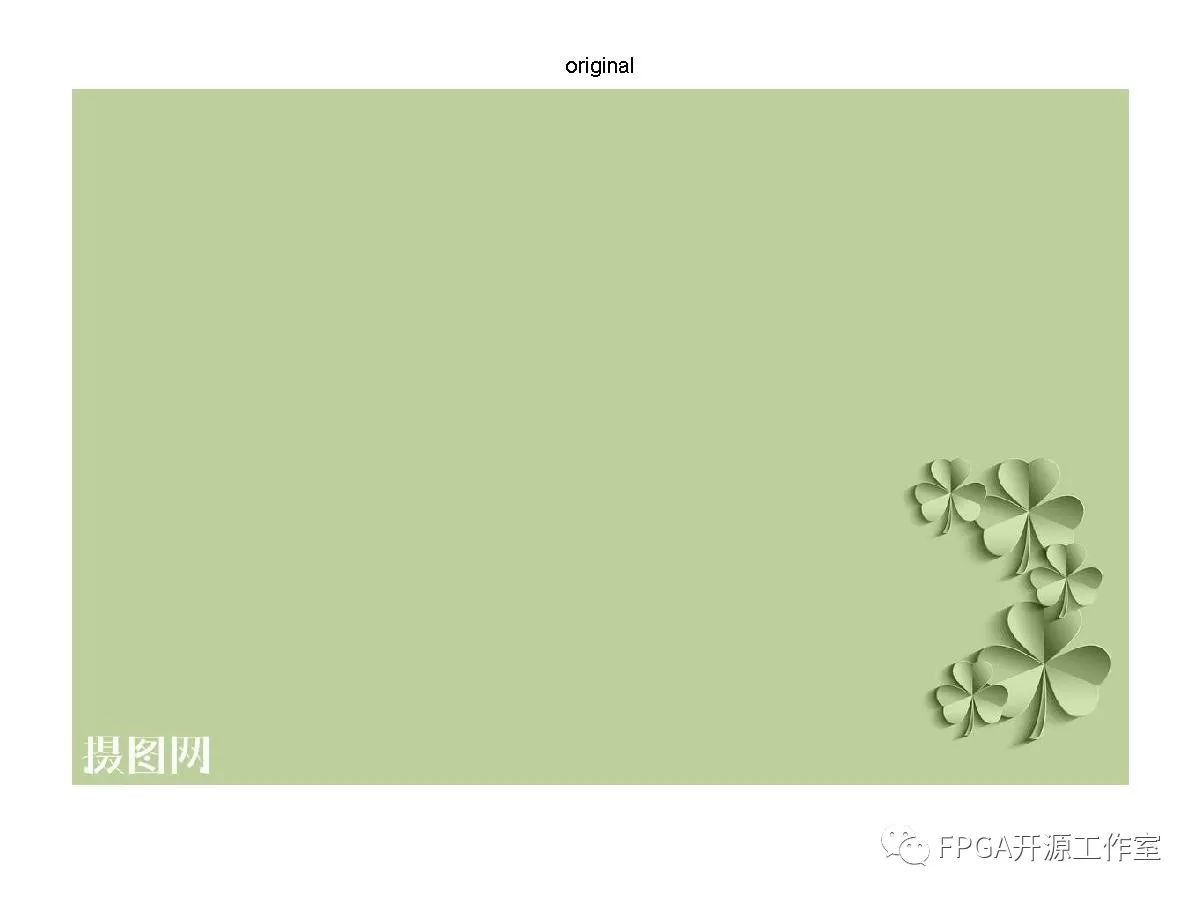原始图像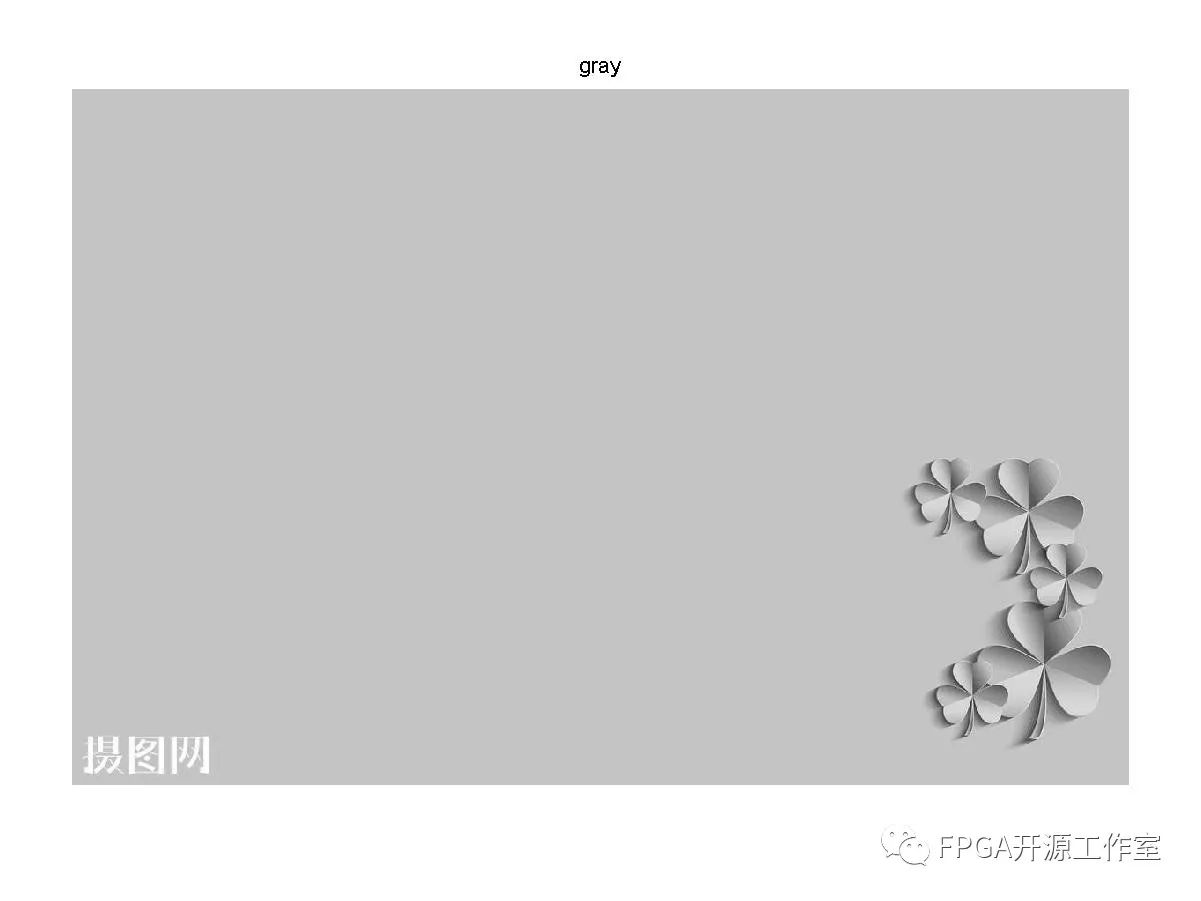灰度图像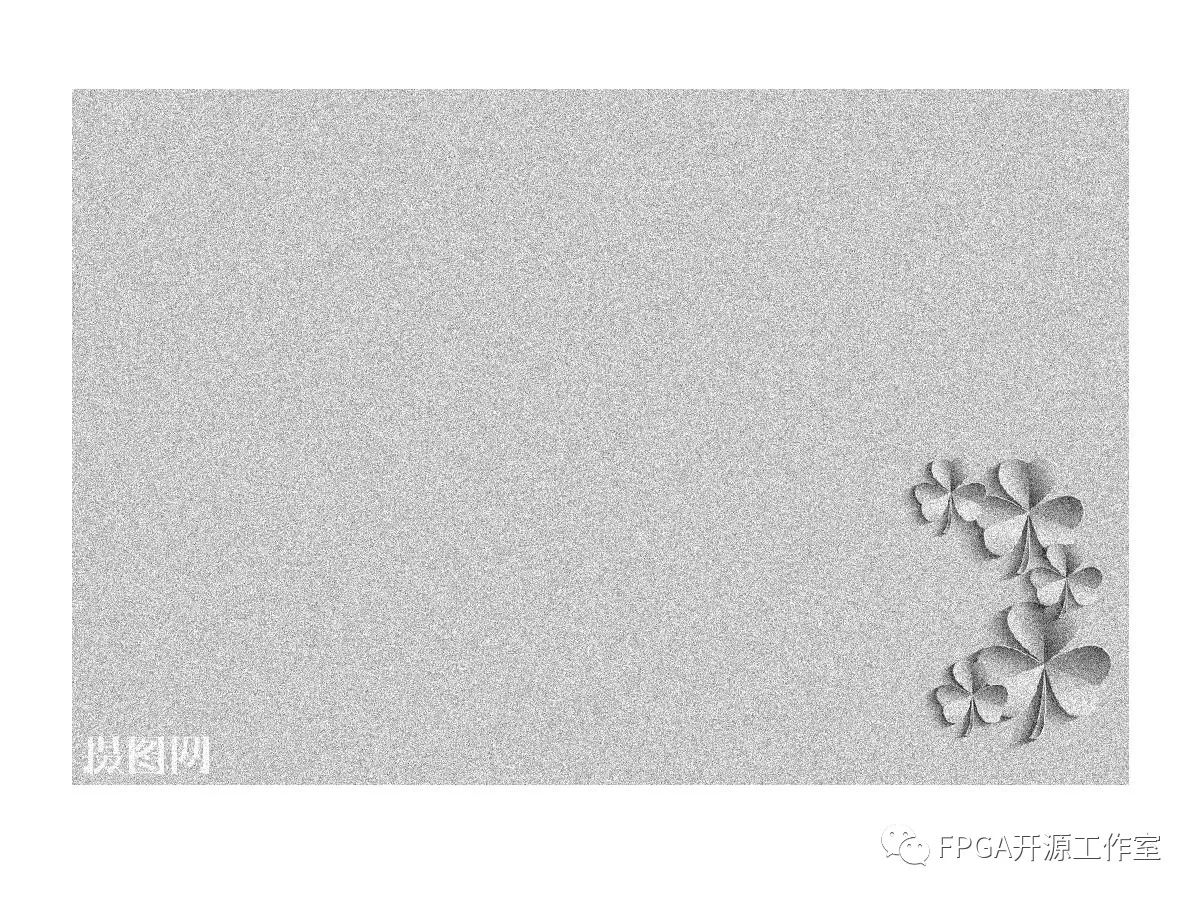加入高斯噪声的灰度图像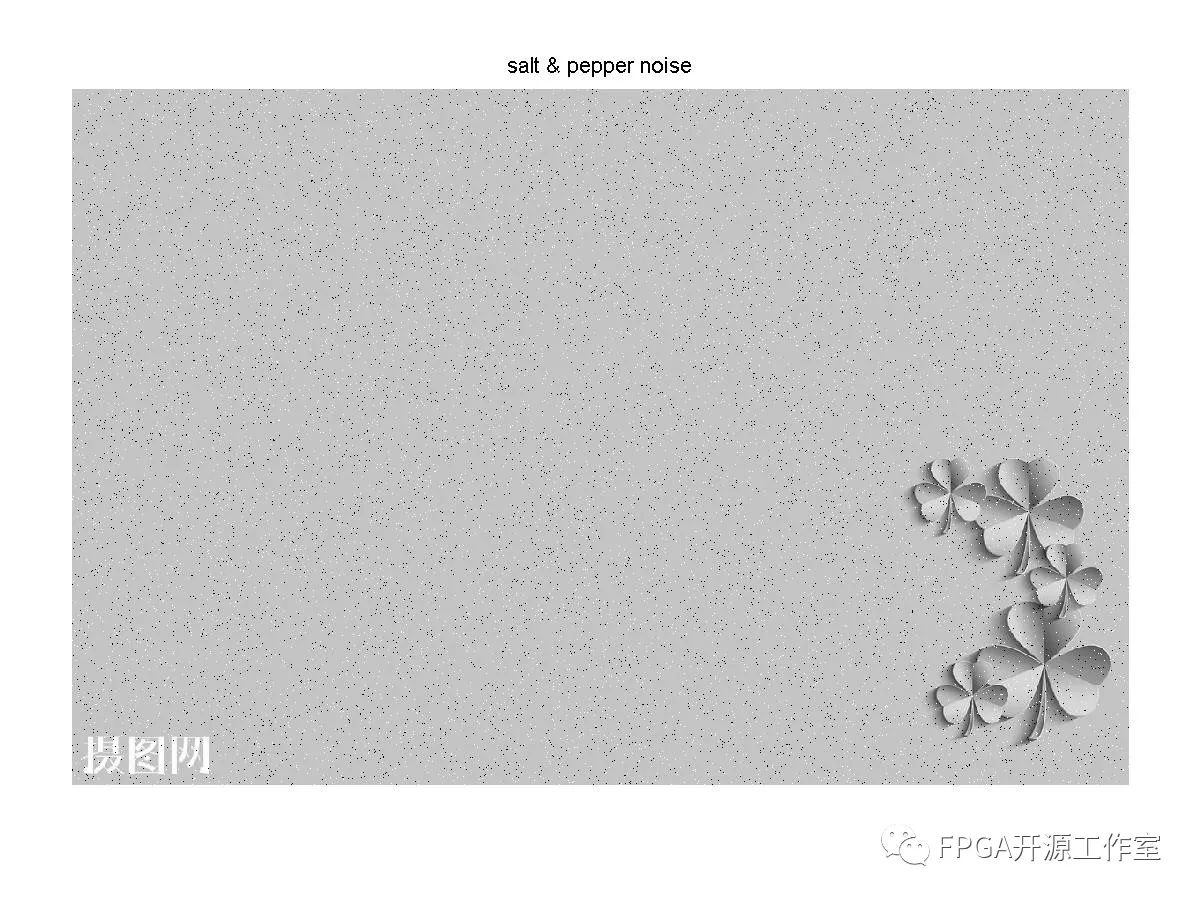加入椒盐噪声的灰度图像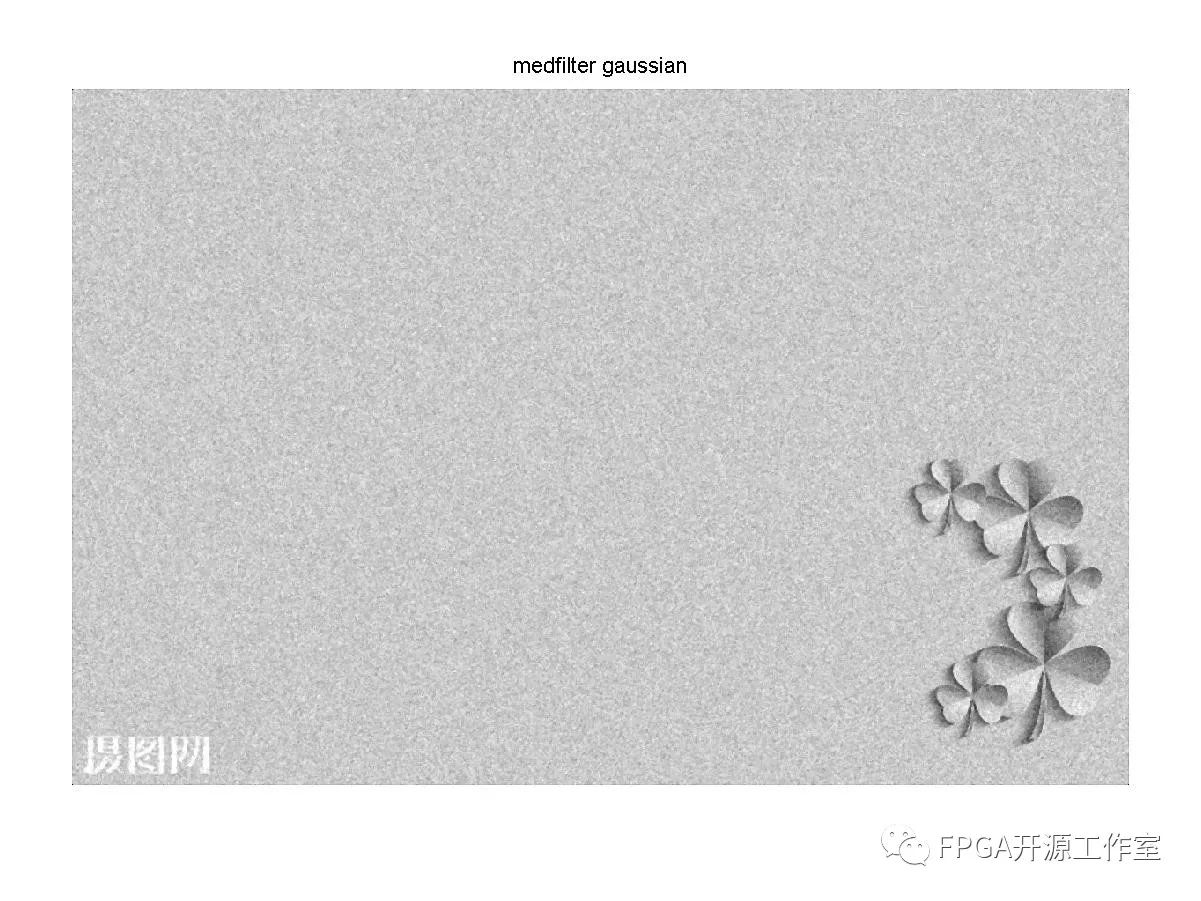经过中值滤波后的高斯噪声灰度图像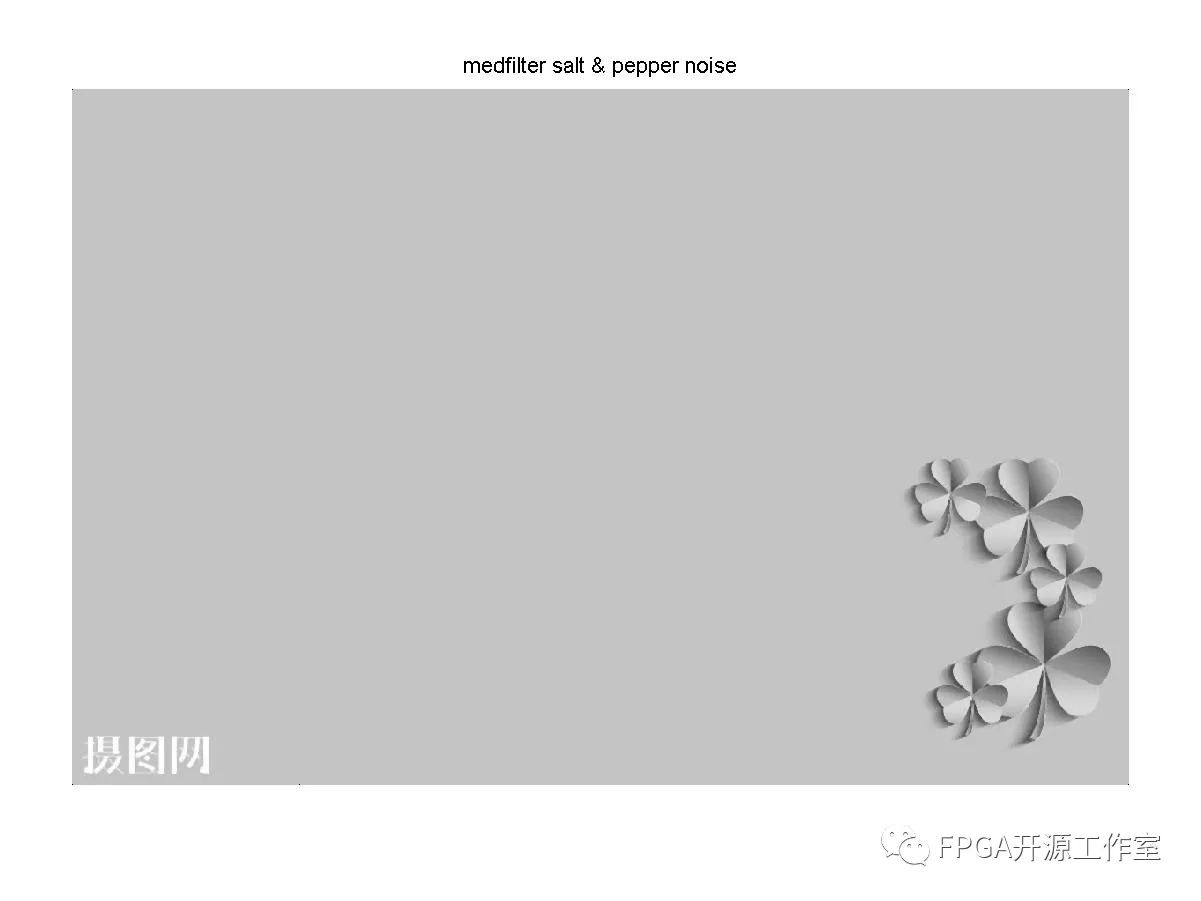经过中值滤波后的椒盐噪声灰度图像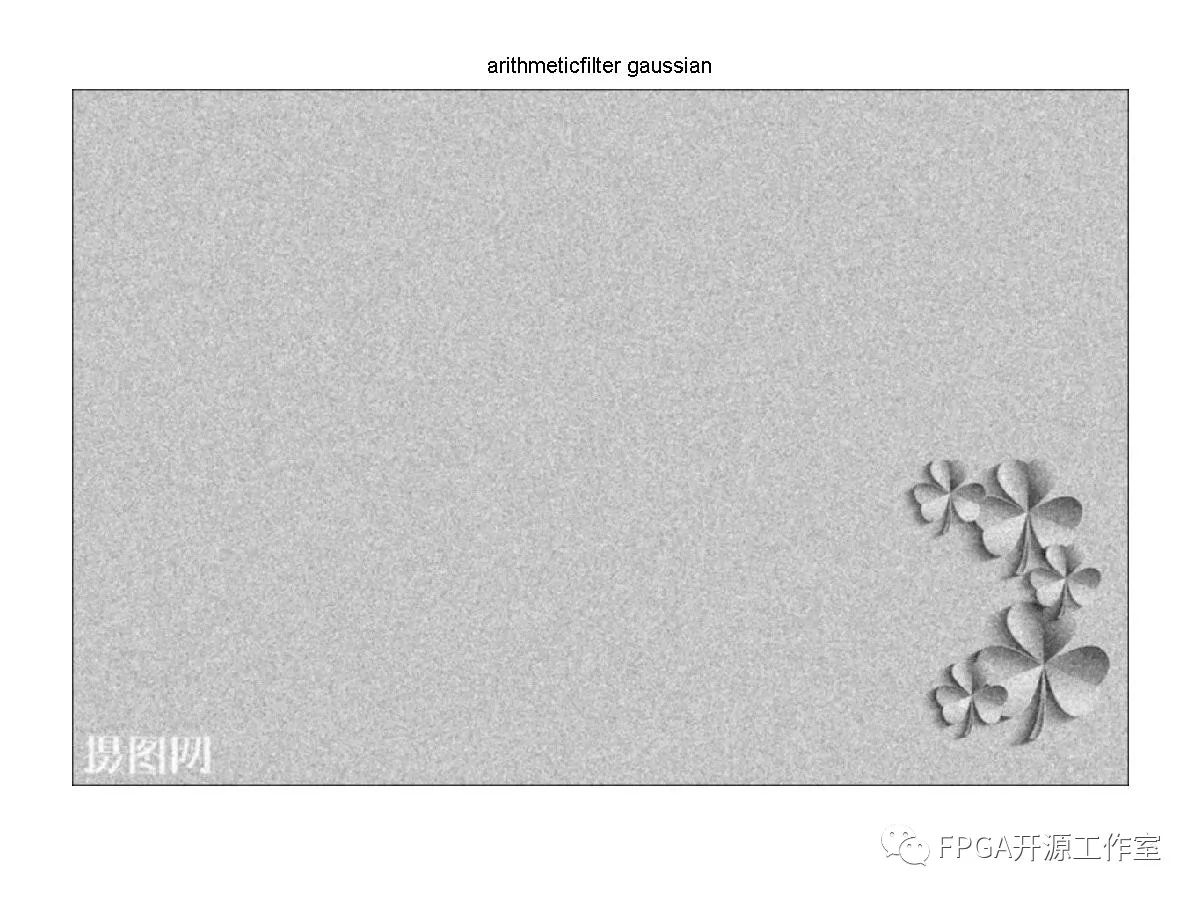经过均值滤波的高斯噪声灰度图像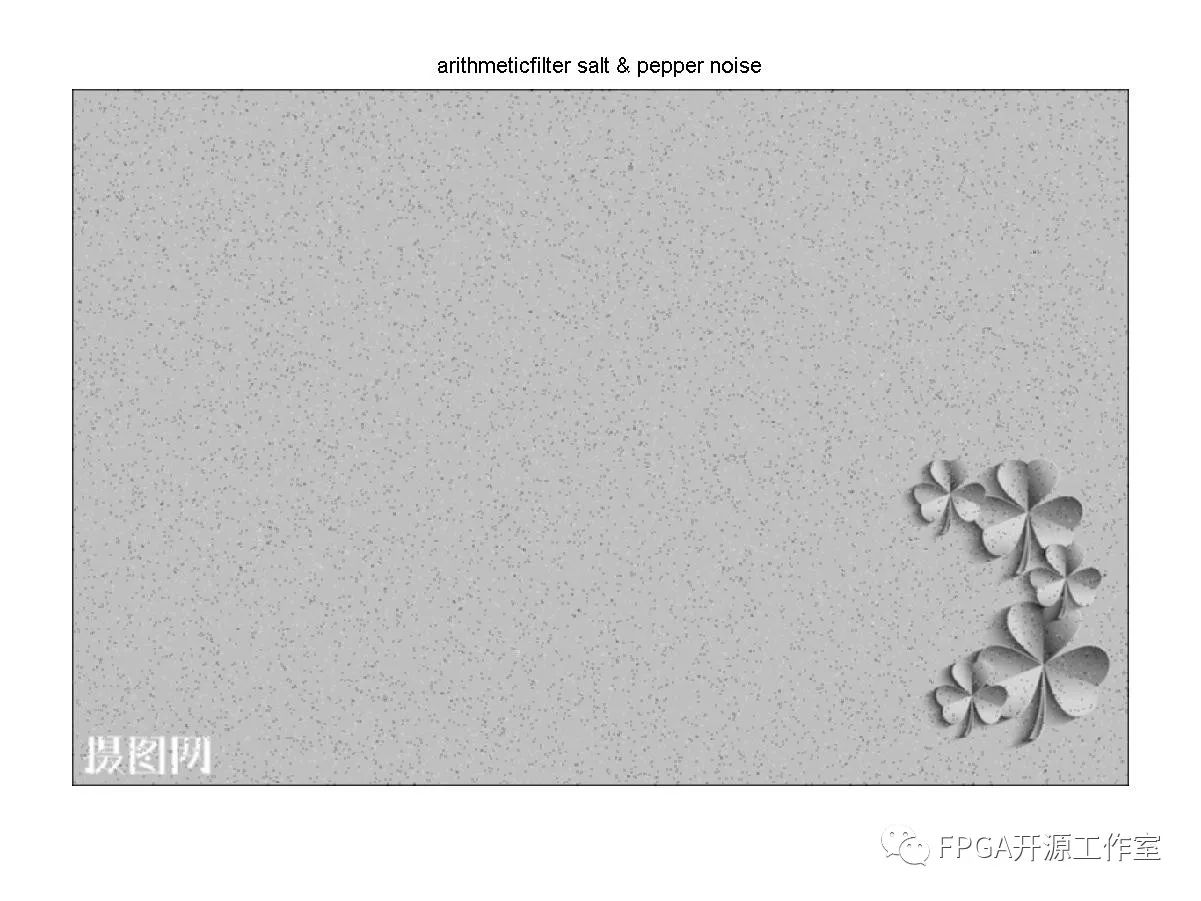经过均值滤波的椒盐噪声灰度图像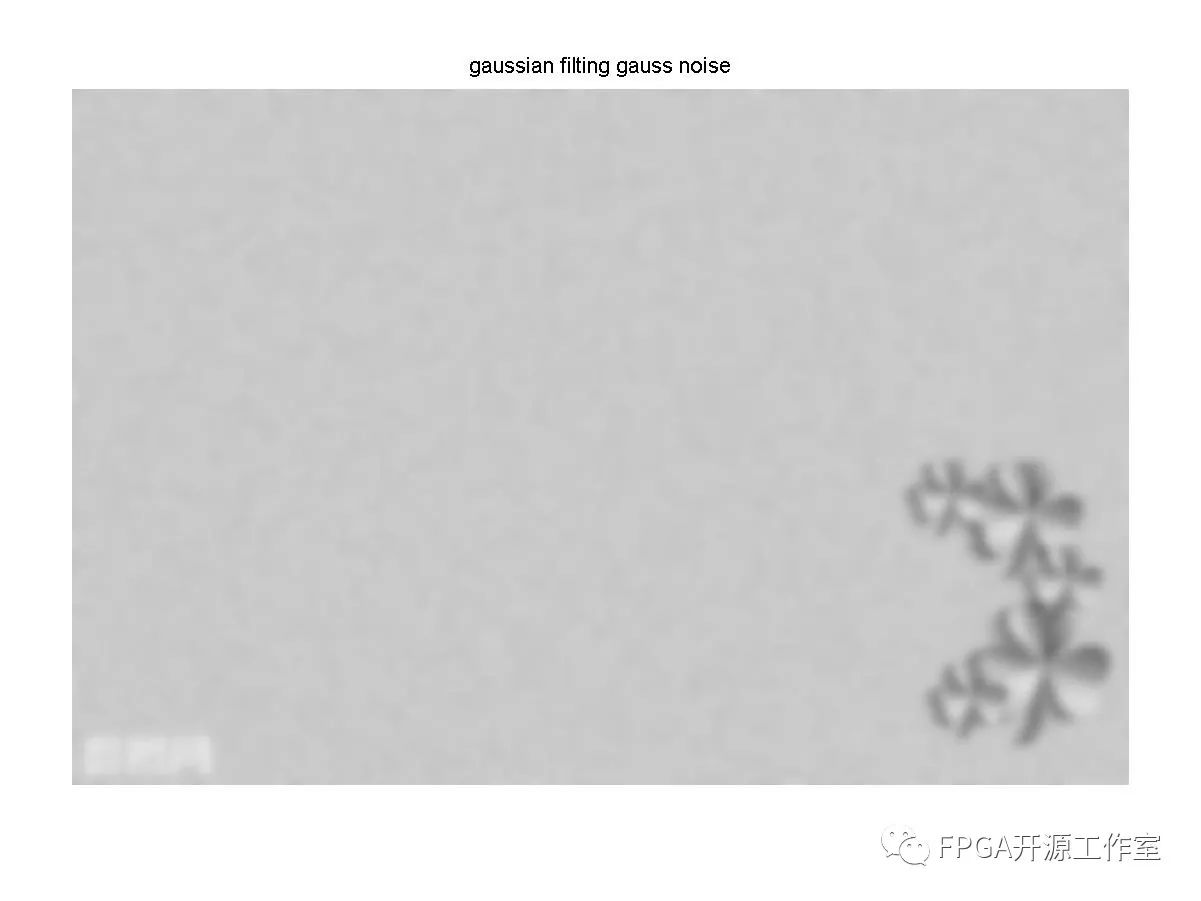经过高斯滤波的高斯噪声灰度图像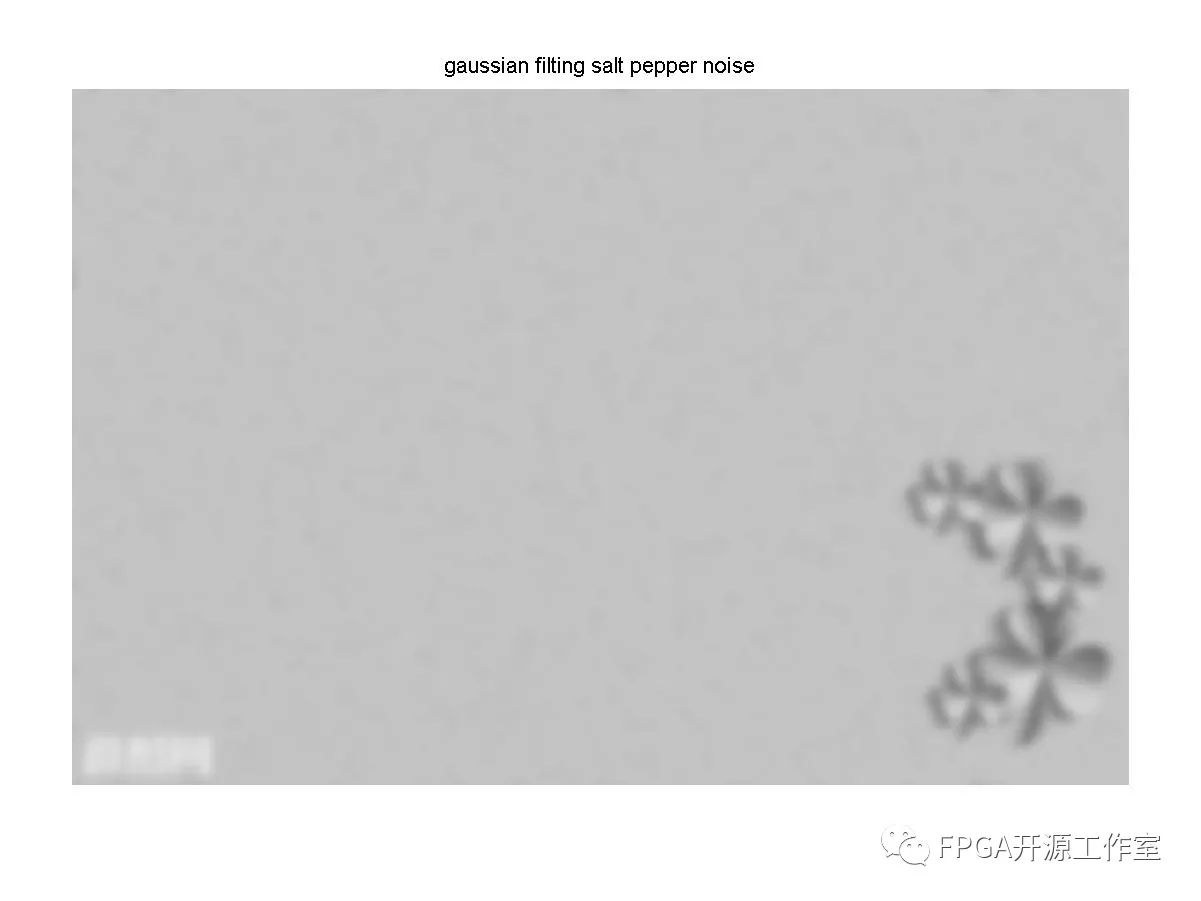经过高斯滤波的椒盐噪声的灰度图像

结果分析：图像经过中值滤波后，高斯噪声没有被完全去除，椒盐噪声几乎被完全去除效果较好。经过均值滤波后不管是高斯噪声还是椒盐噪声大部分都没有被去除，只是稍微模糊化。经过高斯滤波后，高斯噪声和椒盐噪声几乎被很大程度的模糊化，原图好像被加上了一层蒙版。

欢迎大家关注我的微信公众号FPGA开源工作室和资源共享QQ群。(* ￣3)(ε￣ *)

展开全文• 一. 中值滤波： 中值滤波器是一种可以使图像平滑的滤波器。... python实现中值滤波和均值滤波，并用两种滤波器对受到椒盐噪声污染的图像进行去噪 import cv2 import numpy as np # Median fil...

一. 中值滤波：

中值滤波器是一种可以使图像平滑的滤波器。它使用滤波器范围内的像素的中值去代表该范围内所有的像素。中值滤波是消除图像噪声最常见的手段之一，特别是消除椒盐噪声，中值滤波的效果要比均值滤波更好。

二. python实现中值滤波和均值滤波，并用两种滤波器对受到椒盐噪声污染的图像进行去噪

import cv2

import numpy as np

# Median filter

def median_filter(img, K_size=3):

H, W, C = img.shape

tmp = out.copy()

# filtering

for y in range(H):

for x in range(W):

for c in range(C):

return out

# Average filter

def average_filter(img, G=3):

out = img.copy()

H, W, C = img.shape

Nh = int(H / G)

Nw = int(W / G)

for y in range(Nh):

for x in range(Nw):

for c in range(C):

out[G*y:G*(y+1), G*x:G*(x+1), c] = np.mean(out[G*y:G*(y+1), G*x:G*(x+1), c]).astype(np.int)

return out

# Median Filter and Average Filter

out1 = median_filter(img, K_size=3)

out2 = average_filter(img,G=3)

# Save result

cv2.imwrite("out1.jpg", out1)

cv2.imwrite("out2.jpg", out2)

cv2.waitKey(0)

cv2.destroyAllWindows()

三. 实验结果受到椒盐噪声污染的图像 ↑中值滤波后的图像 ↑均值滤波后的图像 ↑

可以明显看出，对于受到椒盐噪声污染的图像，中值滤波往往比均值滤波的去噪效果要好！

四. 参考内容：

展开全文数字图像处理 opencv 中值滤波
• 讨论了中值滤波和均值滤波的去噪性能，在更一般的噪声模型下，当噪声污染的概率较小时，中值滤波抑制噪声的能力优于均值滤波；而当噪声污染的概率较大时，均值滤波抑制噪声的能力优于中值滤波
• 空间域滤波器是最传统最简单的一类图像降噪算法，简单到我都有点不太想做写这篇博客（傲娇了…），但是为了内容的完整性，我还是在此总结下，空间域滤波器可以分为局部滤波器非局部滤波器，其中局部滤波器又分为...

图像降噪算法——中值滤波/均值滤波/高斯滤波/双边滤波

空间域滤波器是最传统最简单的一类图像降噪算法，简单到我都有点不太想做写这篇博客（傲娇了…），但是为了内容的完整性，我还是在此总结下，空间域滤波器可以分为局部滤波器非局部滤波器，其中局部滤波器又分为线性滤波器非线性滤波器。本博客介绍几种比较经典的局部滤波器：中值滤波、均值滤波、高斯滤波、双边滤波。其中均值滤波和高斯滤波为线性滤波器，中值滤波和双边滤波为非线性滤波。

1. 基本原理

因为这些滤波器原理相对都比较简单，因为以我的理解简单描述下：
(1) 中值滤波
以目标像素周围 3 × 3 3\times3 的邻域为例，就是将 3 × 3 3\times3 邻域中九个像素灰度值进行排序，将中间灰度值作为目标像素的灰度值。椒盐噪声影响的像素的灰度值通常都非常大或者非常小，因此通过排序会被消除掉。

(2) 均值滤波
同样以目标像素周围 3 × 3 3\times3 的邻域为例，就是将 3 × 3 3\times3 邻域中九个像素灰度值的平均值作为目标像素的灰度值

(3) 高斯滤波
高斯滤波就是将均值滤波中的平局值改为高斯加权平均值，邻域中年距离目标像素越远的像素灰度值权重越小，通常通过生成一个高斯模板实现

(4) 双边滤波
双边滤波是在高斯滤波的基础上在权重设计中进一步考虑了像素灰度梯度的影响，首先考虑高斯部分权重（下图中的Spatial weight）： w d ( i , j , k , l ) = exp ⁡ ( − ( i − k ) 2 + ( j − l ) 2 2 σ d 2 ) w_{d}(i, j, k, l)=\exp \left(-\frac{(i-k)^{2}+(j-l)^{2}}{2 \sigma_{d}^{2}}\right) 然后考虑像素灰度梯度部分权重（下图中的Range weight）： w r ( i , j , k , l ) = exp ⁡ ( − ∥ f ( i , j ) − f ( k , l ) ∥ 2 2 σ r 2 ) w_{r}(i, j, k, l)=\exp \left(-\frac{\|f(i, j)-f(k, l)\|^{2}}{2 \sigma_{r}^{2}}\right) 其中 ( i , j ) (i,j) 为模板中邻域像素坐标， ( i , j ) (i,j) 为模板中心像素坐标， f ( i , j ) f(i,j) 为坐标 ( i , j ) (i,j) 处的像素值， σ d \sigma_d σ r \sigma_r 分别为两个权重的方差，将两部分权重相乘即获得双边滤波的权重： w ( i , j , k , l ) = w d ( i , j , k , l ) ∗ w r ( i , j , k , l ) = exp ⁡ ( − ( i − k ) 2 + ( j − l ) 2 2 σ d 2 − ∥ f ( i , j ) − f ( k , l ) ∥ 2 2 σ r 2 ) w(i, j, k, l)=w_{d}(i, j, k, l) * w_{r}(i, j, k, l)=\exp \left(-\frac{(i-k)^{2}+(j-l)^{2}}{2 \sigma_{d}^{2}}-\frac{\|f(i, j)-f(k, l)\|^{2}}{2 \sigma_{r}^{2}}\right) 可以参看下图进行理解：2. C++代码实现

下面是基于OpenCV对以上四种滤波器的实现：

Mat Denoise::MedeanFilter(const Mat &src, int size)
{
Mat dst = src.clone();
int start = size/2;
for(int i = start; i < dst.rows-start; i++)
{
for(int j = start; j < dst.cols-start; j++)
{
vector<uchar> model;
for(int m = i-start; m <= i+start; m++)
{
for(int n = j-start; n <= j+start; n++)
{
model.push_back(src.at<uchar>(m,n));
}
}
sort(model.begin(), model.end());
dst.at<uchar>(i,j) = model[size*size/2];
}
}
return dst;
}

//均值滤波
Mat Denoise::MeanFilter(const Mat &src, int size)
{
Mat dst = src.clone();
int start = size/2;
for(int i = start; i < dst.rows-start; i++)
{
for(int j = start; j < dst.cols-start; j++)
{
int sum = 0;
for(int m = i-start; m <= i+start; m++)
{
for(int n = j-start; n <= j+start; n++)
{
sum += src.at<uchar>(m,n);
}
}
dst.at<uchar>(i,j) = (uchar)(sum/size/size);
}
}
return dst;
}

//高斯滤波
Mat Denoise::GaussianFilter(const Mat &src, int size, double sigma)
{
vector<vector<double>> gaussianTemplate = GaussianTemplate(size, sigma);
Mat dst = src.clone();
int start = size/2;
for(int i = start; i < dst.rows-start; i++)
{
for(int j = start; j < dst.cols-start; j++)
{
int sum = 0;
for(int m = i-start; m <= i+start; m++)
{
for(int n = j-start; n <= j+start; n++)
{
sum += src.at<uchar>(m,n)*gaussianTemplate[m-i+start][n-j+start];
}
}
dst.at<uchar>(i,j) = (uchar)sum;
}
}
return dst;
}

vector<vector<double>> Denoise::GaussianTemplate(int size, double sigma)
{
vector<vector<double>> temp;
double base = 1.0 / 2.0 / CV_PI / sigma / sigma;
for(int i = 0; i < size; i++)
{
vector<double> vec;
for(int j = 0; j < size; j++)
{
double a = (pow(i - size/2, 2) + pow(j - size/2, 2)) / 2.0 / sigma / sigma;
double b = base * exp(-a);
vec.push_back(b);
}
temp.push_back(vec);
}
return temp；
}

//双边滤波
Mat Denoise::BilateralFilter(const Mat &src, int size, double sigmaD, double sigmaR)
{
vector<vector<double>> tempD;
vector<double> tempR;
Mat dst = src.clone();

//生成定义域模板
for(int i = 0; i < size; i++)
{
vector<double> vec;
for(int j = 0; j < size; j++)
{
double a = (pow(i - size/2, 2) + pow(j - size/2, 2)) / 2.0 / sigmaD / sigmaD;
double b = exp(-a);
vec.push_back(b);
}
tempD.push_back(vec);
}

//生成值域模板
for(int i = 0; i < 256; i++)
{
double a = (i * i / 2.0 / sigmaR / sigmaR);
double b = exp(-a);
tempR.push_back(b);
}

int start = size/2;
for(int i = start; i < dst.rows-start; i++)
{
for(int j = start; j < dst.cols-start; j++)
{
double sum = 0;
double weightSum = 0;
for(int m = i-start; m <= i+start; m++)
{
for(int n = j-start; n <= j+start; n++)
{
double weight = tempD[m-i+start][n-j+start] * tempR[abs(src.at<uchar>(m,n)-src.at<uchar>(i,j))];
sum += src.at<uchar>(m,n)*weight;
weightSum += weight;
}
}
dst.at<uchar>(i,j) = (uchar)sum/weightSum;
}
}
return dst;
}

运行结果如下：
首先，原图如下图所示：我们首先看看中值滤波椒盐噪声的效果:
添加椒盐噪声后图像如下：中值滤波后效果如下：然后我们来对比下均值滤波高斯滤波双边滤波高斯噪声的效果：
添加高斯噪声的图像如下：均值滤波后效果如下：高斯滤波后效果如下：双边滤波效果如下：3. 结论

1. 对于椒盐噪声，中值滤波的效果是可以接受的，但是不可避免地也带来了边缘模糊的问题。
2. 对于高斯噪声，高斯滤波和双边滤波效果上肉眼看上去可能差不多，但都比均值滤波要好，但是毕竟局部空间域算法比较简单，咱不能要求太高。

有问题欢迎交流~

此外，这里我写一个各种算法的总结目录图像降噪算法——图像降噪算法总结，对图像降噪算法感兴趣的同学欢迎参考

展开全文计算机视觉 图像降噪 数字图像处理
• python调用高斯滤波函数或者中值对大数据集进行滤波PYTHON
• 目录 一、程序 二、仿真结果 三、比较结论 ...%% 图像读取、灰度化与加椒盐噪音 ...%调用函数，处理图像使之灰度化 ...%% 中值滤波 ...%中值滤波函数 %% 均值滤波 h0=1/9.*[1 1 1 1 1 1 1 1 1];%定义平滑模板 p_J

目录

一、程序

二、仿真结果

三、比较结论

一、程序

clear; close all; clc
%% 图像读取、灰度化与加椒盐噪音
I=myreb2gray(I);%调用函数，处理图像使之灰度化
J = imnoise(I,'salt & pepper');%椒盐滤波
%% 中值滤波
z_J=medfilt2(J);%中值滤波函数
%% 均值滤波
h0=1/9.*[1 1 1 1 1 1 1 1 1];%定义平滑模板
p_J=filter2(h0,J);
p_J=uint8(p_J);
%% Image display
figure
subplot(2,2,1),imshow(I);%输出图像
title('原图像')%给原图像加标题
subplot(2,2,2),imshow(J);%输出图像
title('加椒盐噪声后图像')%给原图像加标题
subplot(2,2,3),imshow(z_J);%输出图像
title('中值滤波后图像')%给图像加标题
subplot(2,2,4),imshow(p_J);%输出图像
title('低通滤波后图像')%给图像加标题

二、仿真结果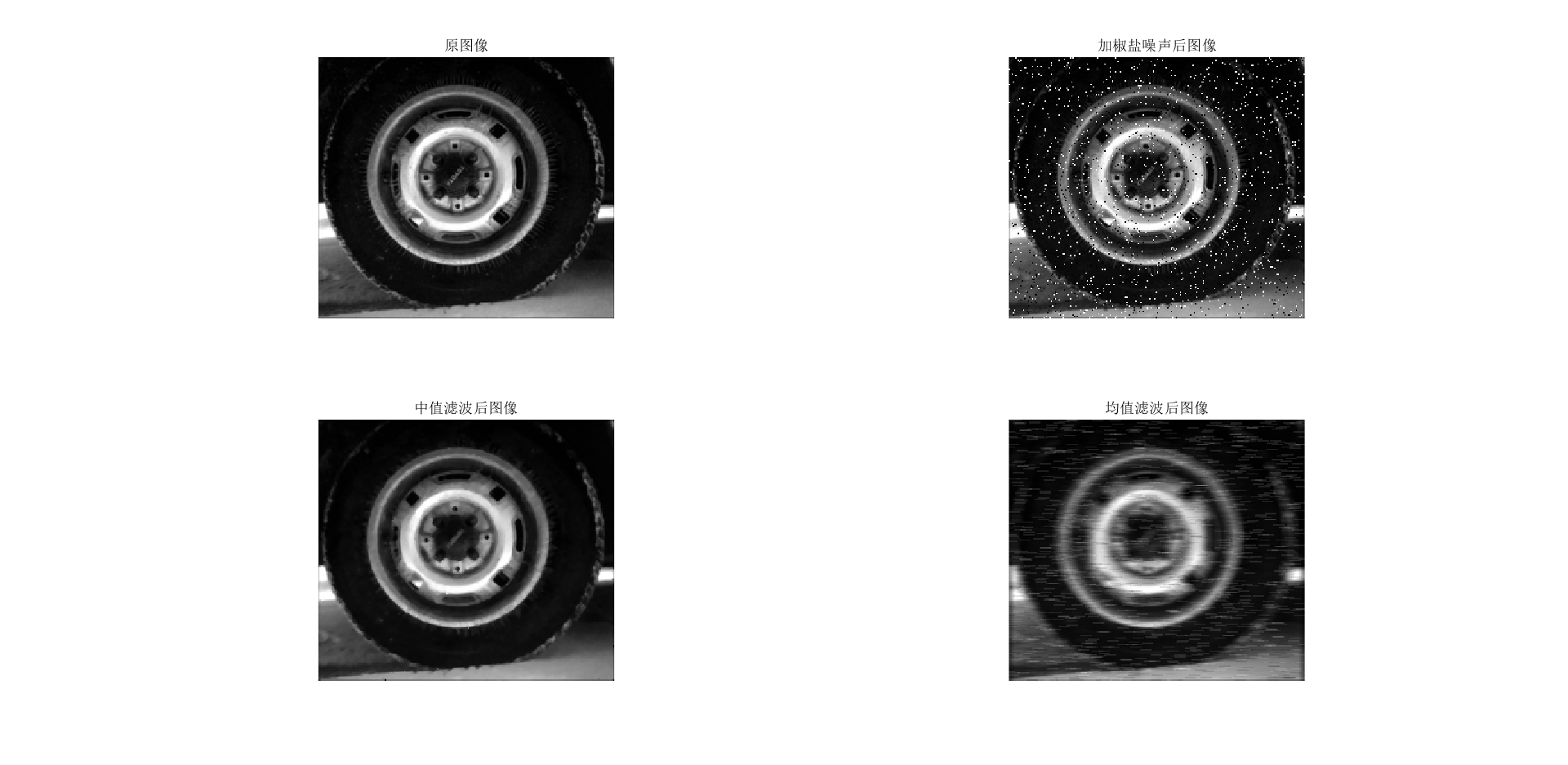三、比较结论

相较于均值滤波而言，中值滤波器更适合处理椒盐噪声。

展开全文matlab 图像处理
• 写在前面HIT大三上学期视听觉信号处理课程中视觉部分的实验一，经过学长们实验的对比发现...实现图像的空域滤波：中值滤波和均值滤波算法，并选取适合的方法对1中的图像进行平滑处理，要求显示处理之后的结果。实...
• 均值滤波和中值滤波的内容非常基础，均值滤波相当于低通滤波，有将图像模糊化的趋势，对椒盐噪声基本无能为力。中值滤波的优点是可以很好的过滤掉椒盐噪声，缺点是易造成图像的不连续性。
• 添加高斯噪声，并用高斯滤波、均值滤波中值滤波、双边滤波四种滤波去噪方式，并且分别得到这四种滤波后的信噪比值，通过比较信噪比值，得到最佳的滤波去噪处理方式。添加噪声的浓度可以改变，并且也可以改变卷积核...Matlab
• 均值滤波 *图像均值滤波 read_image (Image, 'claudia') get_image_size (Image, Width, Height) dev_close_window () ...*生成高斯噪声 gauss_distribution (20, Distribution) add_noise_distributihalcon 图像处理 滤波
• 该算法在自适应中值滤波与斜率差值的基础上，采用图像局部均值与方差的方式对噪声点进行预判定，并对图像边缘进行二次邻域均值滤波。实验结果表明，该算法能够有效去除高密度椒盐噪声，并且能较好地保留细节信息。椒盐噪声
• 图像处理：中值滤波&均值滤波

万次阅读 多人点赞 2018-08-16 10:57:19
转自：openCV之中值滤波&amp;均值滤波（及代码实现）：...均值滤波（及代码实现）首先我们看一下图像滤波的概念。图像滤波，即在尽量保留图像细节特征的条件下对目标图像的噪声进行抑...图像处理 中值滤波 均值滤波
• 高斯滤波 中值滤波均值滤波

千次阅读 2021-02-25 10:27:32
高斯噪声：顾名思义指服从高斯分布（正态分布）的一类噪声，通常是因为不良照明温度引起的传感器噪声。通常在RGB图像中，显现比较明显。如图： 椒盐噪声，通常是由图像传感器，传输通道，解压处理等产生的黑白...图像处理
• 均值滤波 图片中一个方块区域（一般为3*3）内，中心点的像素为全部点像素值的平均值。均值滤波就是对于整张图片进行以上操作。 我们可以看下图的矩阵进行理解 缺陷：均值滤波本身存在着固有的缺陷，即它不...matlab
• I=imread('D:\TuPian\lion.png'); subplot(3,3,1);imshow(I);title('原始图像');... %添加高斯噪声均值为0，方差为0.02 subplot(3,3,4);imshow(G);title('高斯噪声图像'); j1 = fspecial('averamatlab
• 1、中值滤波2113的原理：对于一串连续输入的信号5261(量化后是一组数据)4102。如下图所示，1653是输入的原信号。中值滤波的原理为，重新计算每一个x的输出值(y)，新的输出值。相当于y=new(x)，new的操作是，从在以x...
• 本文是一篇基于python+pyqt的图像处理项目与设计。...在空域图像处理中，常用的去噪方法：均值滤波中值滤波高斯滤波、双边滤波。 （对于正在写python，图像处理等设计论文的人有很大的借鉴作用）opencv qt5 pyqt python 图像处理
• 该滤波边缘使用邻近值填充，结果图像不会出现黑边，中值滤波对椒盐噪声效果较好，均值滤波高斯噪声效果较好，本资源提供了，中值滤波和均值滤波的实现，以及测试代码。图像处理
• 转自:https://blog.csdn.net/zaishuiyifangxym/article/details/89788020 目录 1 图像增强——图像平滑        1.1 图像增强简介   ...2 均值滤...图像 滤波 数字图像处理
• 图像滤波，即在尽量保留图像细节特征的条件下对目标图像的噪声进行抑制，是图像预处理中不可缺少的操作，其处理效果的好坏将直接影响到后续图像处理和分析的有效性可靠性。 图像滤波的主要目的：为了消除图像中...
• 1.图像处理均值滤波中值滤波高斯滤波各自的适用场景；2.OpenCV中均值滤波中值滤波高斯滤波的实现
• https://blog.csdn.net/yanqingan/article/details/5738026
• 线性滤波： 方框滤波、均值滤波高斯滤波 非线性滤波： 中值滤波、双边滤波图像 图像滤波要求： 不能损害图像的轮廓边缘， 图像清晰视觉效果更好 （1）方框滤波 它是滤波器中最简单的，是通过...
• 均值滤波 定义： 均值滤波是典型的线性滤波算法，它是指在图像上对目标像素给一个模板，该模板包括了其周围的临近像素（以目标像素为中心的周围8个像素，构成一个滤波模板，即包括目标像素本身），再用模板中的全体...opencv python...